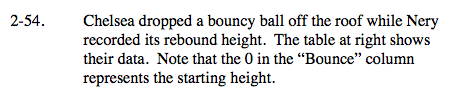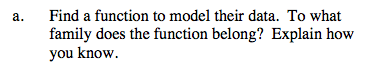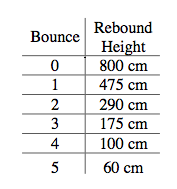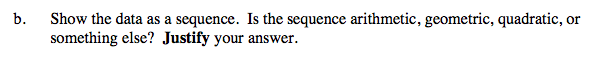Home > A2C > Chapter 2 > Lesson 2.1.4 > Problem2-54

2-54.
1. Chelsea dropped a bouncy ball off the roof while Nery recorded its rebound height. The table below shows their data. Note that the 0 in the “Bounce” column represents the starting height. Homework Help ✎

Bounce

Rebound Height

0

800 cm

1

475 cm

2

290 cm

3

175 cm

4

100 cm

5

60 cm

1. Find a function to model their data. To what family does the function belong? Explain how you know.

2. Show the data as a sequence. Is the sequence arithmetic, geometric, quadratic, or something else? Justify your answer.Look for a multiplier that will approximate the table entries.The function is exponential, because the ratio of one rebound is roughly constant.

t(n) = 800(0.6)nThe function is roughly geometric, even though it is not exact.